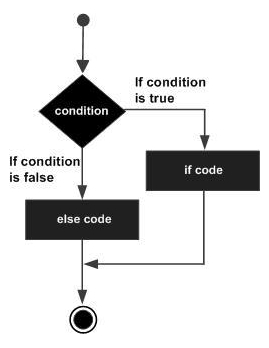# Java if-then-else statement

In Java, if-then-else is represented by the if-else statement.

An if statement can be followed by an optional else statement, which executes when the Boolean expression is false.

## Syntax

Following is the syntax of an if...else statement −

if(Boolean_expression) {
// Executes when the Boolean expression is true
}else {
// Executes when the Boolean expression is false
}

If the boolean expression evaluates to true, then the if a block of code will be executed, otherwise else block of code will be executed.

## Flow Diagram### Example

Live Demo

public class Test {

public static void main(String args[]) {
int x = 30;

if( x < 20 ) {
System.out.print("This is if statement");
}else {
System.out.print("This is else statement");
}
}
}

This will produce the following result −

### Output

This is else statement